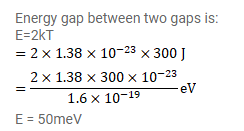# When a semiconducting material is doped with an impurity.

Question:

When a semiconducting material is doped with an impurity. New acceptor levels are created. In a particular thermal collision, a valence electron receives an energy equal to $2 \mathrm{kT}$ and just reaches one of the acceptor levels. Assuming that the energy of the energy of the electron was at the top edge of the valence band and that the temperature $T$ is equal to $300 \mathrm{k}$, find the energy of the acceptor levels above the valence band.

Solution: